# 游戏模拟——Position based dynamics

PBD是一种省略了速度层的直接作用于位置的控制方法，方法的主要优点是它的可控性、性能、数值稳定，在游戏开发领域应用很广，近年的UE chaos底层也采用了PBD方法。

# Verlet积分

verlet积分在游戏开发领域应用也很广泛，并且PBD论文中提到了PBD的思想和verlet有相近之处，这里也顺便介绍一下。

## 基本积分方法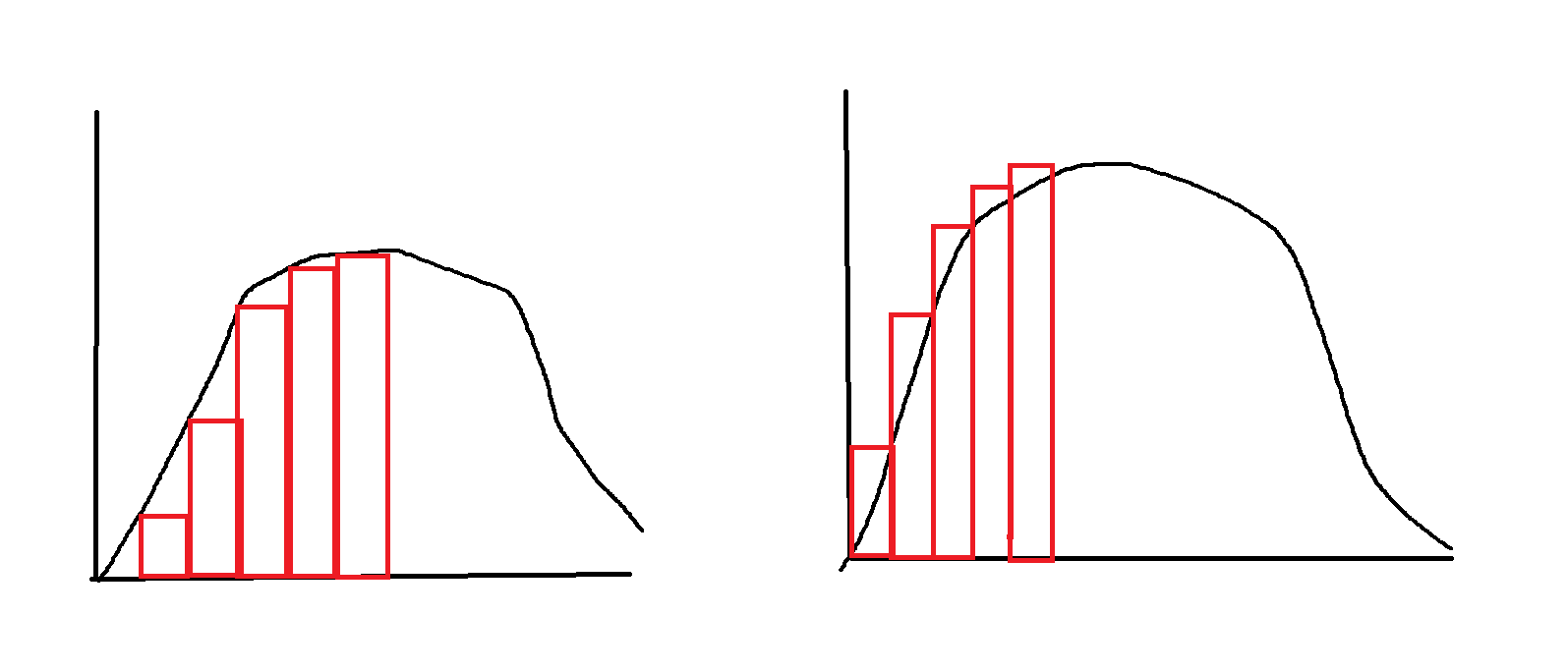## Verlet 算位置

verlet积分是一种牛顿运动方程的积分方法，他的积分方法有着良好的数值稳定性，对比简单的欧拉方法没有显著的额外计算成本。

$x _{t+\Delta t} = x_t + v(t)\Delta t + \frac{1}{2}a(t)\Delta t^2 + \frac{1}{6}b(t)\Delta t^3 + O(\Delta t^4)$

$x _{t-\Delta t} = x_t - v(t)\Delta t + \frac{1}{2}a(t)\Delta t^2 - \frac{1}{6}b(t)\Delta t^3 + O(\Delta t^4)$

$x _{t+\Delta t} = 2 x_t - x _{t-\Delta t} + a(t)\Delta t^2 + O(\Delta t^4)$

## Verlet 算速度

$x(t+\Delta t)−x(t−\Delta t)=2v(t)\Delta t+ \frac{1}{3}b(t)\Delta t^3$

$v(t) = \frac {x(t+ \Delta t) - x(t - \Delta t)}{2\Delta t} + \frac{b(t)\Delta t^3}{3\Delta t}$

$v(t) = \frac {x(t+ \Delta t) - x(t - \Delta t)}{2\Delta t} + O(\Delta t^2)$

$v(t+\Delta t) = \frac {x(t+ \Delta t) - x(t)}{\Delta t} + O(\Delta t)$

$\frac {x(t+\Delta t)−x(t)}{\Delta t} =v(t)+ \frac{1}{2}a(t)\Delta t + \frac{1}{6}b(t)\Delta t^2$

wiki百科里则提供了一种更加高精度的速度推算方法叫 velocity verlet ，利用梯形法（头尾值加起来求平均，之前的小矩形可以想象成梯形来看）算的加速度来推算速度，让速度的精度到达二阶，至于为什么是二阶，因为梯形法的结果是二阶精度，这个精度貌似可以传递。

$v(t+\Delta t) = v(t) + \frac {a(t)+a(t+ \Delta t)}{2} \Delta t$

a的$$t+\Delta t$$是一般来说是一个和位置有关的函数，先求了位置，接下来这里面都是已知量了。

# PBD

## 基于力的方法解碰撞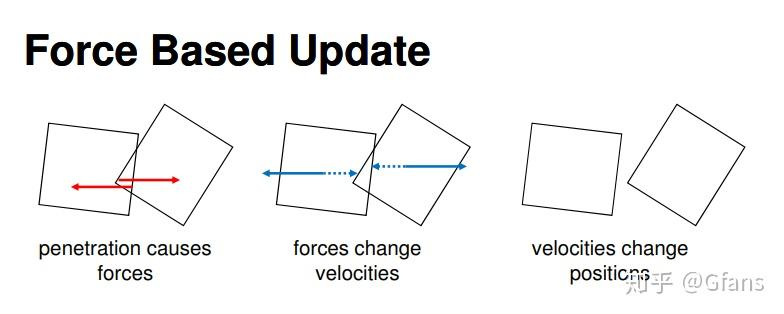### 过冲问题

1、两刚体相交过深导致计算出来的力太大，求解的初始条件残差太大
2、时间步长太大
3、高频振荡：在求解涉及高频振荡的 ODE 时，显式方法可能难以准确捕获这些振荡，从而导致超调。在这种情况下，使用对刚性系统更稳定的隐式积分方法会有所帮助。
4、误差累积：使用显式方法时，误差会随着时间的推移而累积，从而导致显着的超调。

## 基于位置的方法解碰撞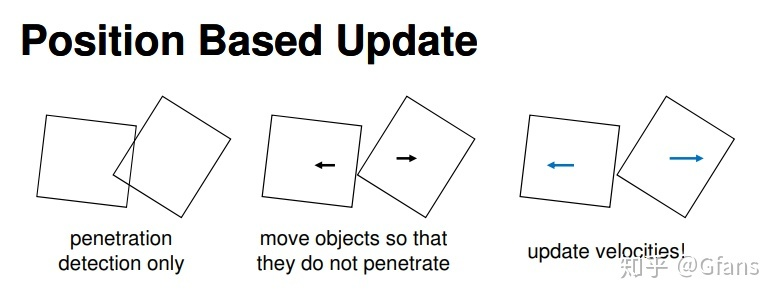## 算法流程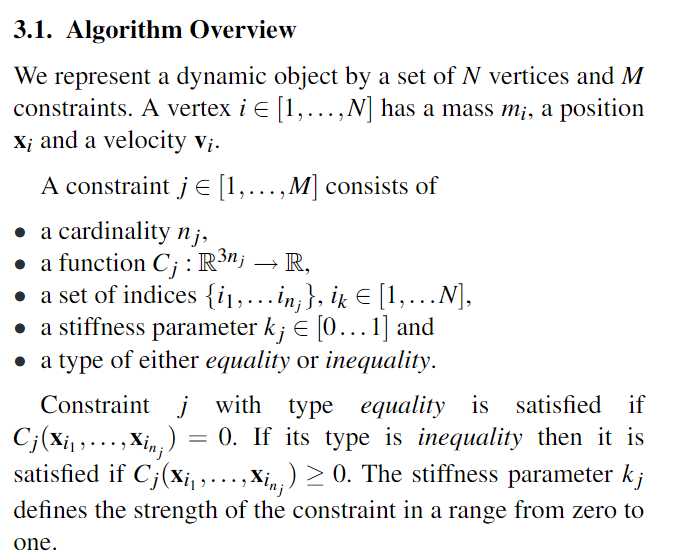• 定义一个约束影响的顶点数量是nj，可以理解为第 j个约束所影响的顶点数目为nj
• 定义约束函数C 顶点数目为3nj，位置信息为xyz，所有的数据总量就是3nj，约束函数就是读取这个3nj的数据算出一个实数
• 约束函数影响的顶点的索引，nj个索引
• 定义这个约束的刚度值
• 定义这个约束是等式约束和不等式约束
等式约束比较强硬，要满足约束函数等于0，不等式约束则是约束函数大于等于0就行，kj定义了这个约束的刚度，刚度越大这个约束要越快被满足。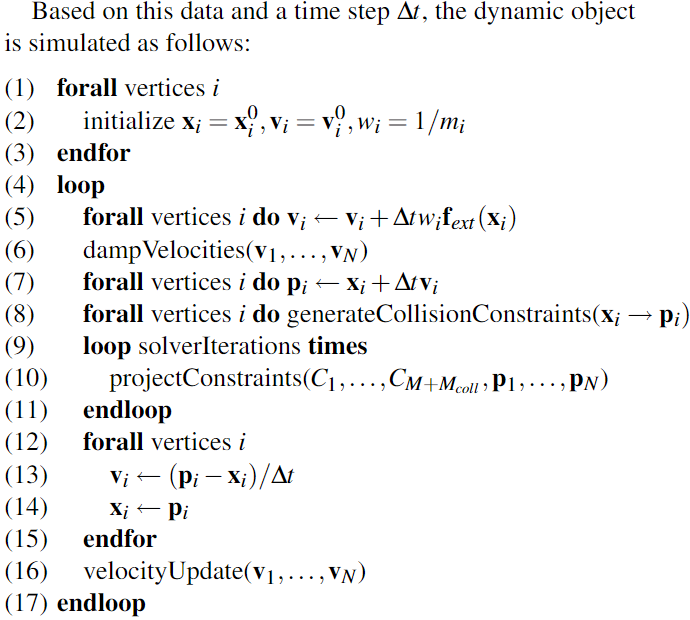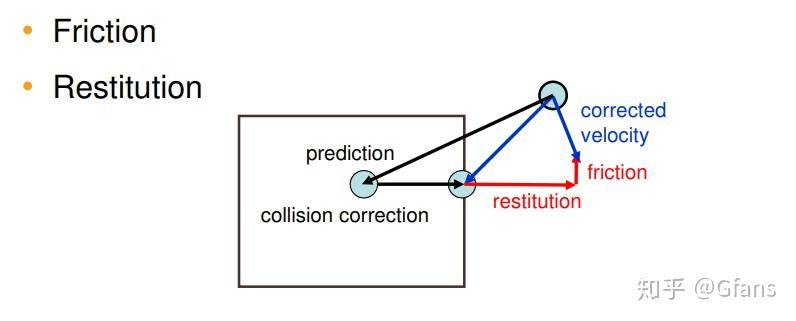## 求解器借用的思想

$C = |p_1-p_2| - d$

## 关于动量守恒

PBD方法里提到了动量守恒的话题，PBD本身方法的流程他是想说明按照他的流程走，只要约束函数定义时候是动量守恒的，那么结果是动量守恒的。

## 约束投影

$C(p+\Delta p) = 0$

$C(p+\Delta p) \approx C(p) + \nabla_p C(p) \cdot \Delta p$

$\Delta p = \lambda \nabla_p C(p)$

$\Delta p = - \frac{C(p)}{|\nabla_pC(p)|^2} \nabla_p C(p)$

$\Delta p_i = - s \nabla_{p_i} C(p)$

$s = \frac{C(p)}{\sum_j {|\nabla_{p_j}C(p) |^2}}$

delta P1则表示delta P向量的第一点分量，是一个3分量的向量，其实就是第一个点的位移，他应该保证和约束函数在这个第一个点的偏导数保证方向相同。

## 简单约束举例

https://zhuanlan.zhihu.com/p/48737753

## 刚度体现

$\Delta p (1-k)^{n_s}$

$k' = 1-(1-k)^{\frac{1}{n_s}}$

$\Delta p (1-k')^{n_s} = \Delta p(1-k)$

flyingziming
2023.4.7

posted @ 2023-04-06 18:40  飞翔的子明  阅读(444)  评论(0编辑  收藏  举报